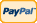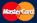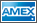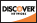•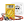I need to measure...
•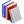I need to calculate...
•Electronics, electrical engineering
•Engineering physics
•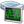Generators
•History events
•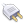Interfaces
•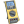Multimeters
•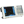Oscilloscopes
•Persona
•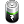Power supplies
•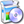Software
•Standards
•Thesaurus
•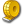Units of measurement

Filter by first letter

# Hall effect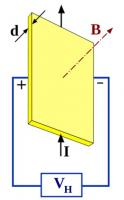The Hall effect is the production of a voltage difference (the Hall voltage) across an electrical conductor, transverse to an electric current in the conductor and a magnetic field perpendicular to the current. It was discovered by Edwin Hall in 1879.

The Hall coefficient is defined as the ratio of the induced electric field to the product of the current density and the applied magnetic field. It is a characteristic of the material from which the conductor is made, since its value depends on the type, number, and properties of the charge carriers that constitute the current.

For a simple metal where there is only one type of charge carrier (electrons) the Hall voltage VH is given by

where I is the current across the plate length, B is the magnetic field, d is the depth (thickness) of the plate, e is the electron charge, and n is the charge carrier density of the carrier electrons.www.wikipedia.org

Back to the list

Rating

Measurement History Events
 Yesterday Today Tomorrow
06/04/1896Units Converter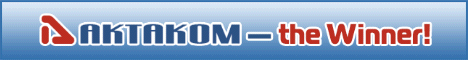Site map|Privacy policy|Terms of Use & Store Policies|How to Buy|Shipping|Payment|© T&M Atlantic, Inc., 2010-2023